Browse by Topic
Related Topics

# New in the Wolfram Language: GreenFunction and Applications in Electricity, ODEs, and PDEsPicture of Green’s Windmill by Kev747 at the English language Wikipedia.

In 1828, an English corn miller named George Green published a paper in which he developed mathematical methods for solving problems in electricity and magnetism. Green had received very little formal education, yet his paper introduced several profound concepts that are now taught in courses on advanced calculus, physics, and engineering. My aim in writing this post is to give a brief biography of this great genius and provide an introduction to GreenFunction, which implements one of his pioneering ideas in Version 10.4 of the Wolfram Language.

George Green was born on July 14, 1793, the only son of a Nottingham baker. His father noticed young George’s keen interest in mathematics, and sent him to a local school run by Robert Goodacre, a well-known science popularizer. George studied at Goodacre Academy between the ages of eight and nine, and then went to work in his father’s bakery. Later he ran a corn mill built by his father in Sneinton, near Nottingham. He is said to have hated his work at the bakery and the corn mill, and regarded it as annoying and tedious. In spite of his onerous duties, George appears to have continued studying mathematics in his spare time, retreating to the top floor of the 16-meter-high mill, shown above, for this purpose. In 1828, he published the results of his rigorous self-study in “An Essay on the Application of Mathematical Analysis to the Theories of Electricity and Magnetism,” one of the most influential mathematical papers of all time.

Green’s paper of 1828 introduced the potential function, which is well known to students of physics. He also proved a form of Green’s theorem from advanced calculus in this paper. Finally, he introduced the notion of a Green’s function that, in one form or another, is familiar to students of engineering, and is the theme for this post. By sheer chance, Sir Edward Bromhead, a founder of the Analytical Society, purchased and read a copy of Green’s paper. With his encouragement, Green entered Gonville and Caius College in Cambridge University at the age of forty, and eventually became a fellow of the college. He continued to publish papers until his untimely death in 1841, possibly due to lung complications arising from his work at the corn mill. Sadly, recognition for his mathematical work had to wait until 1993, when a plaque was dedicated to his memory in Westminster Abbey. Today, the Green’s Mill and Science Centre in Nottingham carries on the work of promoting George Green’s reputation as one of the greatest scientists of his age.

I will now give an introduction to GreenFunction using concrete examples from electrical circuits, ordinary differential equations, and partial differential equations.

The basic principle underlying a Green’s function is that, in order to understand the response of a system to arbitrary external forces, it is sufficient to understand the system’s response to an impulsive force of the DiracDelta type.

As an illustration of the above principle, consider a circuit that is composed of a resistor R and an inductor L, and is driven by a time-dependent voltage v[t], as shown below: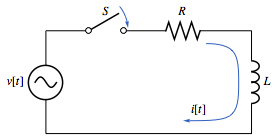The current i[t] in the circuit can then be computed by solving the differential equation:

L i´(t)+R i(t)==v(t)

Let’s assume that the voltage source is a battery supplying a unit voltage. Next, suppose that you close the switch S for a fleeting moment at time t = s and then quickly throw it open again. The current induced in the circuit by this impulsive action can be computed by applying GreenFunction to the left-hand side of the above differential equation: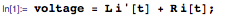The initial value of the current is assumed to be zero, since the switch was open until time t = s: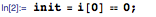Here is the result given by GreenFunction for this example: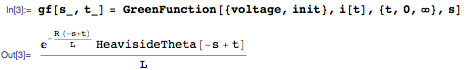The following plot for s = 1 shows that the current is 0 for all times t < 1, then rises instantaneously to its peak value at t = 1, and finally decreases to 0 with the passage of time: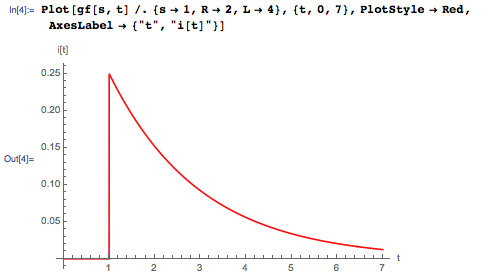The behavior of the circuit in the above situation is usually called its impulse response, since it represents the response of the circuit to an impulsive voltage.

Next, suppose that you close the switch at time t = 0 and leave it closed at all later times. Thus the voltage steps up from its initial value 0 to a constant value 1, and can be modeled using the HeavisideTheta function: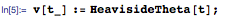The step voltage can be visualized as follows: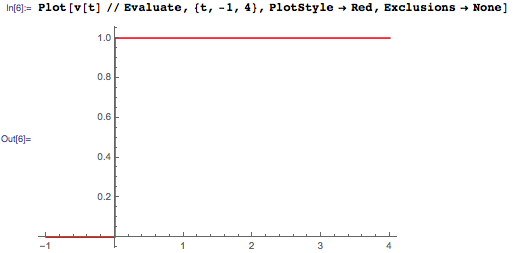You can now compute the current in the circuit by performing the following integral involving the voltage and the Green’s function: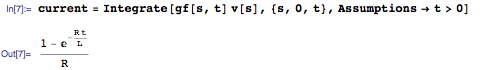The integral computed above is essentially a weighted sum of the Green’s function with the voltage source at all times s prior to a given time t, and is called a convolution integral.

As the plot below shows, the current for the step voltage source gradually increases from its value 0 at t = 0 to a steady-state value: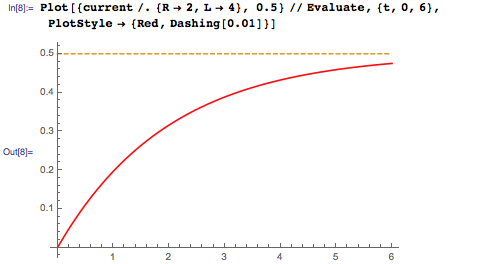The behavior of the circuit in the above situation is usually called its step response, since it represents the response of the circuit to a step voltage.

Finally, suppose that the voltage source supplies an alternating voltage—for example: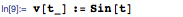You can once again compute the current in the circuit by performing a convolution integral of the voltage with the Green’s function, as shown below: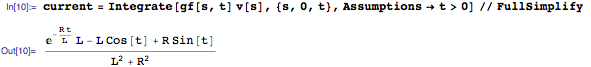You can also obtain the result using DSolveValue as follows: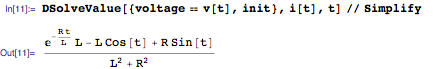As the plot below shows, the current settles down to a steady alternating pattern for large values of the time: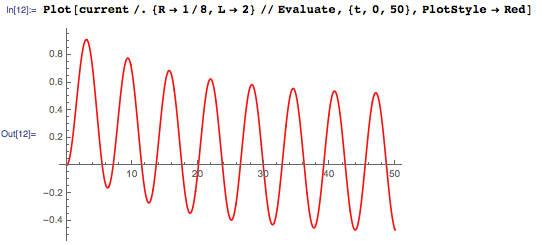To summarize, the Green’s function encodes all the information that is required to study the response of the circuit to any external voltage. This magical property makes it an indispensable tool for studying a wide variety of physical systems.

The two-step procedure for solving the differential equation associated with a circuit, which I discussed above, can be applied to any linear ordinary differential equation (ODE) with a forcing term on its right-hand side and homogeneous (zero) initial or boundary conditions. For example, suppose you wish to solve the following second-order differential equation: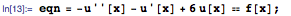Assume that the forcing term is given by: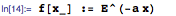Also, suppose that you are given homogeneous boundary conditions on the interval [0,1]: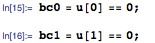As a first step in solving the problem, you compute the Green’s function for the corresponding differential operator (left-hand side) of the equation: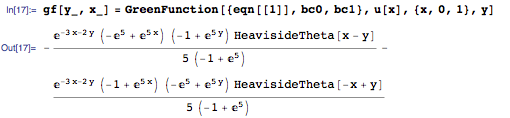The following plot shows the Green’s function for different values of y lying between 0 and 1. Each instance of the function satisfies the zero boundary conditions at both ends of the interval: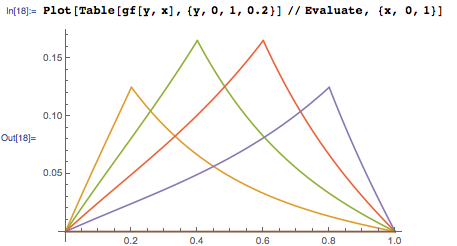You can now compute the solution of the original differential equation with the given forcing term using a convolution integral on the interval [0,1], as shown below: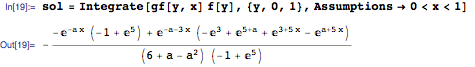Here is a plot of the solution, which shows that it satisfies the homogeneous boundary conditions for different values of the parameter a: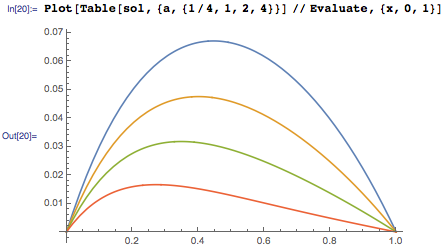Green’s functions also play an important role in the study of partial differential equations (PDEs). For example, consider the wave equation that describes the propagation of signals with finite speed, and that I discussed in an earlier post. In order to compute the Green’s function for this equation in one spatial dimension, use the wave operator (left-hand side of the wave equation), which is given by: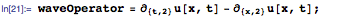Here, x denotes the spatial coordinate that ranges over (-∞,∞), t denotes the time that always ranges over [0,∞), and u[x,t] gives the displacement of the wave at any position and time.

You can now find the Green’s function for the wave operator as follows: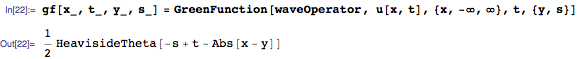The following plot of the Green’s function shows that it becomes 0 outside a certain triangular region in the xt plane, for any choice of y and s (I have chosen both these values to be 0). This behavior is consistent with the fact that the wave propagates with a finite speed, and hence signals sent at any time can only influence a limited region of space at any later time: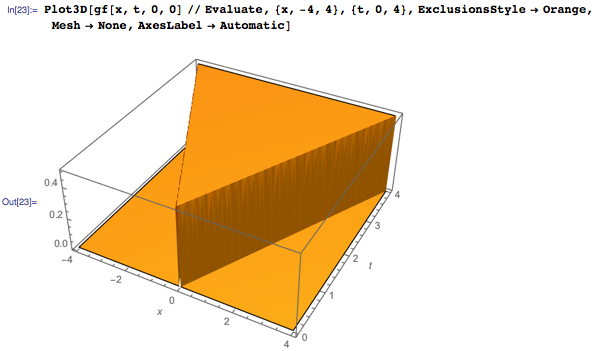The Green’s function obtained above can be used to solve the wave equation with any forcing term, assuming that the initial displacement and velocity of the wave are both zero. For example, suppose that the forcing term is given by: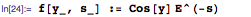You can solve the wave equation with this forcing term by evaluating the convolution integral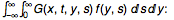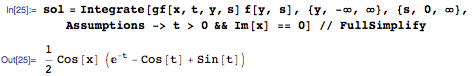The following plot shows the standing wave generated by the solution: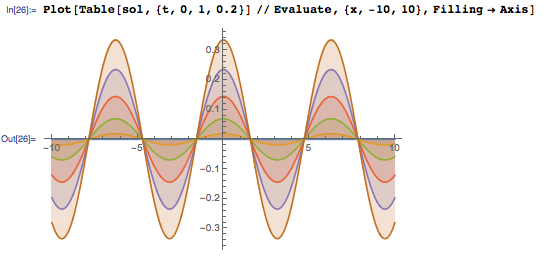Finally, I note that the same solution can be obtained by using DSolveValue with homogeneous initial conditions, as shown below: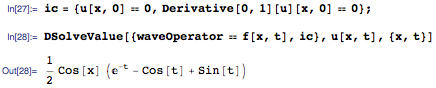Green’s functions of the above type are called fundamental solutions and play an important role in the modern theory of linear partial differential equations. In fact, they provided the motivation for the theory of distributions that was developed by Laurent Schwartz in the late 1940s.

The ideas put forward by George Green in his paper of 1828 are stunning in their depth and simplicity, and reveal a first-rate mind that was far ahead of the times during which he lived. I have found it very inspiring to study the life and work of this great mathematician while implementing GreenFunction for Version 10.4 of the Wolfram Language.